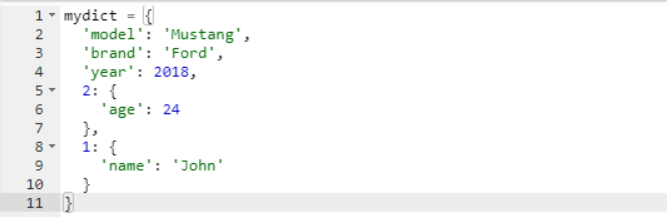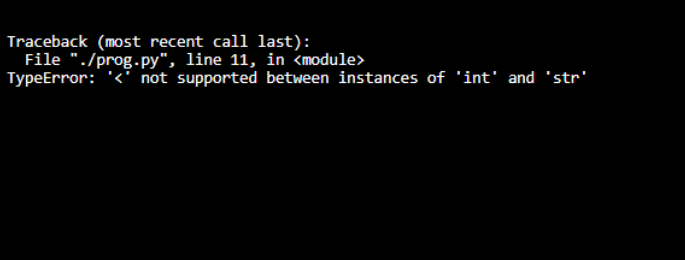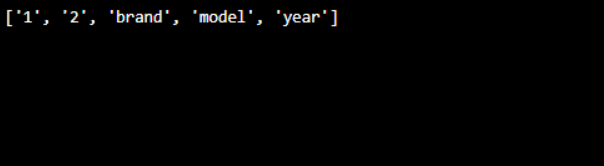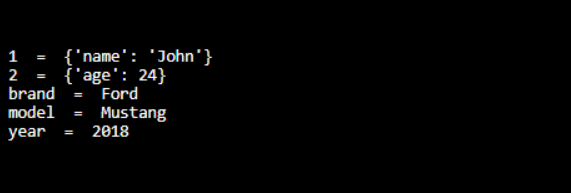## Description

Sometimes there may be a need to access or simply print a python dictionary in a particular order of its keys i.e. ascending or descending as it was in my case. Although it seems that it is not a big issue and that is true in the case of simple data in a dictionary, it can be more tricky with some complicated data in a dictionary.

### Example

Let’s understand it with a simple example. Suppose we have a dictionary with mixed indices i.e. string as well as an integer as given below:Now, if we will try to print the sorted keys using the following piece of code:
print(sorted (mydict.keys()))

It will give us the following error:This is because it is internally trying to compare the string with an integer.

### Solution

So here is the solution for how to convert dictionary keys into a string to perfectly sort it in Python. To resolve this problem, we need to convert all the keys of the dictionary into the string and we can do that through these simple steps:

Step 1: We will create a recursive method with the following code:

```def convertKeysToString(dictionary): """Recursively converts dictionary keys to strings.""" if not isinstance(dictionary, dict): return dictionary return dict((str(k), convertKeysToString(v)) for k, v in dictionary.items())```

Step2: Now, just pass your dictionary by calling this method and you will get the required dictionary with all keys as a string. So if you just print all the keys in sorted order it works:
mydict = convertKeysToString(mydict)
print(sorted (mydict.keys()))Now, if required in any loop with the sorted key, you can also process this dictionary in the following way:
for i in sorted (mydict.keys()) :
print(i, ” = “, mydict[i])

Output: